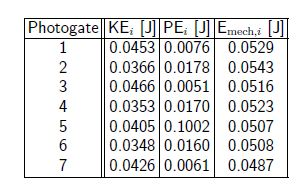# Potential Energy: Mathematical Solution

Example: A steelball with mass in = 0.44 kg and radius r = 1.0 cm moves along a rollercoaster. Seven photogates are placed along the track at different heights hi from the base of the track; we measure the time intervals Δti for the steel ball to pass through each photogate,Calculate the gravitational potential energy, kinetic energy, and total mechanical energy.

Solution: We have the gravitational potential energy PEi = mghi. The (average) speed of the ball is vi = 2r/Δti, as it passes through each photogate. The kinetic energy then follows KE = mvi2/2. Note that the steel ball moves along the track (translational motion), but also rotates [rotational motion]. As a result, the kinetic energy of the ball has a contribution from rotation. In this situation, the contribution of rotational kinetic energy can be described by an “effective” mass m*, greater than the mass in. If the track has a width w = 0.95 cm, we find,Discussion: The mechanical energy of the system (slightly) decreases due to friction.